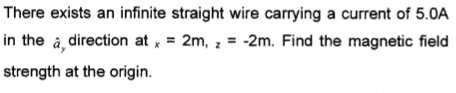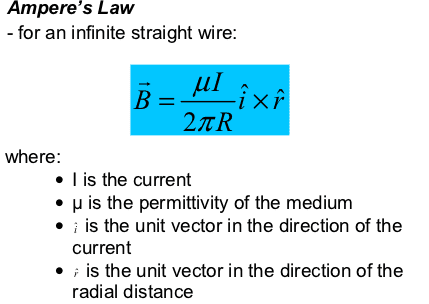# Magnetic Field Strength

wheybags

## Homework Statement## Homework Equations## The Attempt at a Solution

I let µ be 9, as that's the approximate permittivity of air/ free space.
I assumed that as a hat had a subscript y, it ran parallel to the y axis, and worked from there, getting the cross product of the unit vector in the direction of a hat, (which I took to be (0,1,0) ) and (sqrt(1/2), 0, sqrt(1/2)), which I worked out from the x and z positions given, and then scalar multiplying by the preceding stuff.
Did I approach this correctly? If not, where did i go wrong?

## Answers and Replies

Homework Helper
I let µ be 9, as that's the approximate permittivity of air/ free space.

µ is the permeability of free space.

ehild

wheybags
Is permeability the same as permittivity? Beacuse my notes say permittivity.

Mentor
Permeability of vacuum: $$\mu_0 = 4 \pi 10^{-7} N/A^2$$

Permittivity of vacuum: $$\epsilon_0 = \frac{1}{c^2 \mu_0} \approx 8.854 \times 10^{-12} F/m$$

wheybags
So my notes are wrong?

da_nang
No, the permeability is used in magnetic fields, while permittivity is used in electric fields. Permittivity measures the two-way interaction between electric fields and mediums. Like say how much an exterior electric field affects the formation of interior electric fields in water.
Permeabiity on the other hand, measures the capability of a medium to uphold a magnetic field, no matter if an exterior magnetic field creates it or the medium itself.

EDIT: Yes, I'm afraid your notes are wrong.

wheybags
Well, ****, I knew my lecturer was bad, but I mean, really...

wheybags
Forgot to say, if I were to fix that error, was my solution otherwise correct?

Homework Helper
In this problem, the radial vector points from the wire to the origin. So your r-hat vector is not correct.

ehild

wheybags
Oh, I thought it was from the point (in this case the origin) to the wire. Was my interpretation of a hat subscript y correct?

Homework Helper
The current in the wire causes the magnetic field, and R and r-hat is with respect to the wire which is not at the origin now.
The unit vector pointing in the direction of the current is OK.

ehild